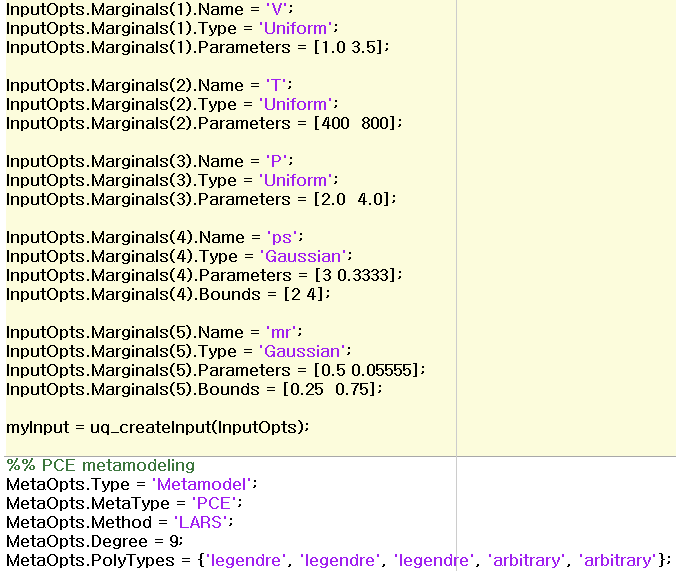# Arbitrary PCE and input moments

I understand that arbitrary polynomial Chaos(aPC) has the advantage of being able to create a PCE model only through input moment even if the input distribution is not functional formation.
In other words, when aPC declares input variable, moments is assumed to be gaussian, uniform, etc., and ‘basis’ is the basis of aPC and is it set to fit into that variable?In other words, in the picture below, is it gPC from first to third, and aPC from fourth and fifth?

Hi @Chemicaleng,

In UQLab, arbitrary PCE is not computed through the moments as suggested by Oladyshkin&Nowak (2012), but through the Stieltjes procedure as suggested by Wan&Karniadakis (2006). You can find the explanation in the PCE user manual in Section 1.3.1.2. Some useful context on arbitrary PCE is given in Torre et al (2019): Data-driven polynomial chaos expansion for machine learning regression.

Note that you need an input model to compute the PCE – it is not enough to specify `.PolyTypes` only. If you have existing data with an unknown distribution, you can use the inference module to infer the distribution. I would recommend to follow the suggestions by Torre et al (2019).

Good luck!Figures are attached excluding the input variable. I’m sorry.
As shown in the figure, the input moment and V, T, P that declared the basis for it are gPC, and ps and mr are aPC assuming that they have gaussian moment. Is it correct?Hi @Chemicaleng,

Are you asking whether it is correct to set `MetaOpts.PolyTypes` to `arbitrary` if the input has a truncated Gaussian distribution, such as in your example the fourth and fifth input variable? The answer would be yes - UQLab would even do it automatically, i.e., you don’t need to specify `PolyTypes` explicitly (see Section 2.4.1 of the PCE user manual).

I saw that paper ‘Data-driven polynomial chaos expansion for machine learning regression’, in that paper, aPC can train the regression model through input moments (not to the input PDF directly), it means, (in above figure) V, T, P is gPC, ps, mr is aPC?
(=That is, if i set the basis, is it gPC, otherwise, is it aPC?)

[ variable V, T, P is declared through Uniform distribution and Legendre polynomial(specify basis function),variable ps, mr is declared through gaussian distribution and arbitrary polynomial ]

I guess you refer to the explanation in section 2.3.2 of “Data-driven polynomial chaos expansion for machine learning regression”. Here they explain the three possibilities of how to deal with non-classical input distributions.

This is the third option they mention, but in the end of that paragraph, they say

An accurate estimation of moments of higher order, however, requires a large number of input samples, thus effectively limiting its applicability in the settings considered here . For this reason, we opt instead for the second approach outlined above.

So in that paper (and in UQLab), the second approach is used instead.

In UQLab you always have to specify an input distribution. If you don’t specify `MetaOpts.PolyTypes`, the PCE type is automatically decided per marginal: if it belongs to the classical families (see Section 1.3.1.1 of the PCE user manual), the corresponding orthonormal polynomials are used (gPC). If not, arbitrary PCE are computed. In particular, a truncated Gaussian distribution does not belong to the classical families. If you want to override this behavior (e.g. use Hermite polynomials for all marginals regardless of their distributions), you can do so using `MetaOpts.PolyTypes`.

So, to summarize: in your case, specifying

``````MetaOpts.PolyTypes = {'legendre', 'legendre', 'legendre', 'arbitrary', 'arbitrary'}
``````

is fine, but unnecessary: this is exactly what UQLab would do internally.